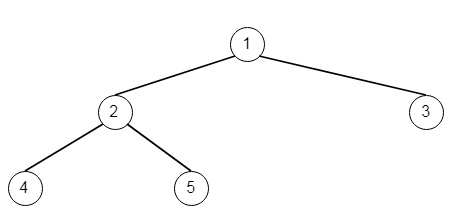# Lowest Common Ancestor of Deepest Leaves in Python

Suppose we have a rooted binary tree, we have to return the lowest common ancestor of its deepest leaves. We have to keep in mind that −

• The node of a binary tree is a leaf node if and only if it has no children

• The depth of the root of the tree is 0, and when the depth of a node is d, the depth of each of its children is d+1.

• The lowest common ancestor of a set S of nodes in the node A with the largest depth such that every node in S is in the subtree with root A.

If the input is [1,2,3,4,5],then the output will be [2,4,5]

To solve this, we will follow these steps −

• Define a method called solve(), this will take node, this will work as follows −

• if node is not present, then return a list with [0, None]

• if left and right subtrees are empty of node, then return a list with [1, None]

• d1, l := solve(left of node), d2, r := solve(right of node)

• if d1 > d2 , then return a list with values [d1 + 1, l]

• otherwise when d2 > d1, then return a list with values [d2 + 1, r]

• return a list with values [d1 + 1, node]

• In the main method, we will perform −

• list := solve(root)

• return list

## Example(Python)

Let us see the following implementation to get a better understanding −

Live Demo

class TreeNode:
def __init__(self, data, left = None, right = None):
self.data = data
self.left = left
self.right = right
def insert(temp,data):
que = []
que.append(temp)
while (len(que)):
temp = que
que.pop(0)
if (not temp.left):
if data is not None:
temp.left = TreeNode(data)
else:
temp.left = TreeNode(0)
break
else:
que.append(temp.left)
if (not temp.right):
if data is not None:
temp.right = TreeNode(data)
else:
temp.right = TreeNode(0)
break
else:
que.append(temp.right)
def make_tree(elements):
Tree = TreeNode(elements)
for element in elements[1:]:
insert(Tree, element)
return Tree
def print_tree(root):
#print using inorder traversal
if root is not None:
print_tree(root.left)
print(root.data, end = ', ')
print_tree(root.right)
class Solution(object):
return self.solve(root)
def solve(self,node):
if not node:
return [0,None]
if not node.left and not node.right:
return [1,node]
d1,l = self.solve(node.left)
d2,r = self.solve(node.right)
if d1>d2:
return [d1+1,l]
elif d2>d1:
return [d2+1,r]
return [d1+1,node]
ob = Solution()
root = make_tree([1,2,3,4,5])
print_tree(ob.lcaDeepestLeaves(root))

## Input

[1,2,3,4,5]

## Output

4, 2, 5,